# Quick And Easy Understanding Of Trigonometry For Class 10

###### ByRamraj Saini###### Synopsis

Trigonometry is introduced in NCERT Class 10 Maths along with applications. Since it is an important chapter not just for the board exams but also from the JEE point of view, knowing quick and easy tips and tricks for trigonometry can be a great help. Here are tips to easily study the many formulae and identities with the help of examples and previous board exam question papers.###### Synopsis

Trigonometry is introduced in NCERT Class 10 Maths along with applications. Since it is an important chapter not just for the board exams but also from the JEE point of view, knowing quick and easy tips and tricks for trigonometry can be a great help. Here are tips to easily study the many formulae and identities with the help of examples and previous board exam question papers.

Most of you have studied triangles. One of them is the right angled triangle. To understand how right triangles are formed, take a look at the example below

• If you visit a tall structure like the Qutub Minar and look at the top as given in the diagram below, you will realise that a right triangle is formed. One interesting question here would be - can a student find out the height of the Minar without measuring it?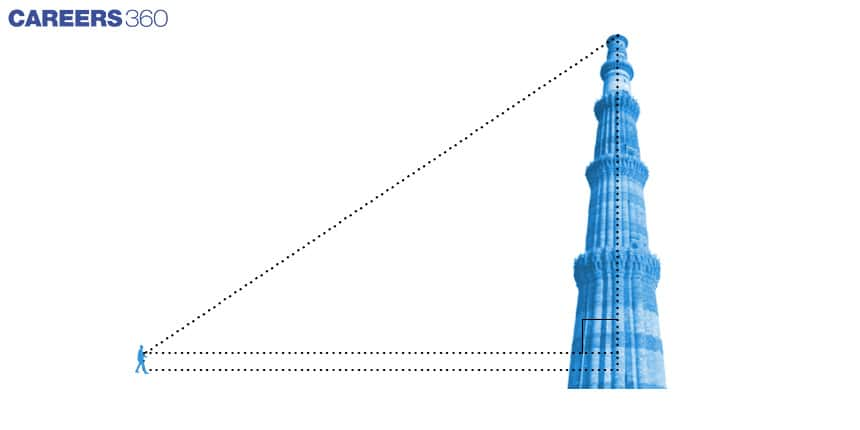• Think about a hot air balloon flying in the air. A girl spots the balloon and runs to tell her mother about it. When her mother comes out of the house to look at the balloon, it would have travelled some distance from point A to point B as shown in figure below. Can one find the altitude of A and B from the ground?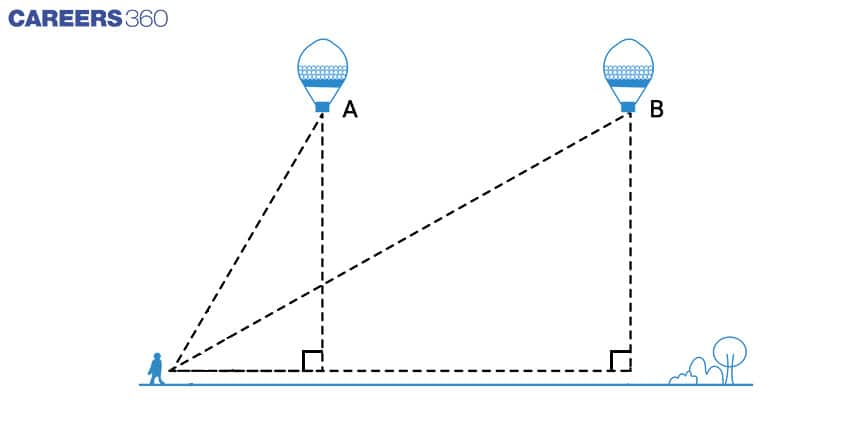In both cases, the answer is yes. Mathematical techniques that can be used to gauge the height or altitude or distance come under “Trigonometry”, which is a branch of mathematics.

Interesting Fact: Trigonometry was used in ancient times to calculate the distances between planets and stars from Earth.

What is Trigonometry?

The study of the relationship between the angles and sides of a triangle is known as trigonometry. Many concepts in engineering and physical sciences are based on trigonometric concepts.

In this article, we will study some trigonometric ratios of a right triangle with respect to its acute angle. Further, we will calculate some trigonometric ratios for some specific angles. We will also determine the trigonometric ratios for angles of measure 0° and 90° and establish some trigonometric identities involving these ratios.

## Trigonometric Ratios: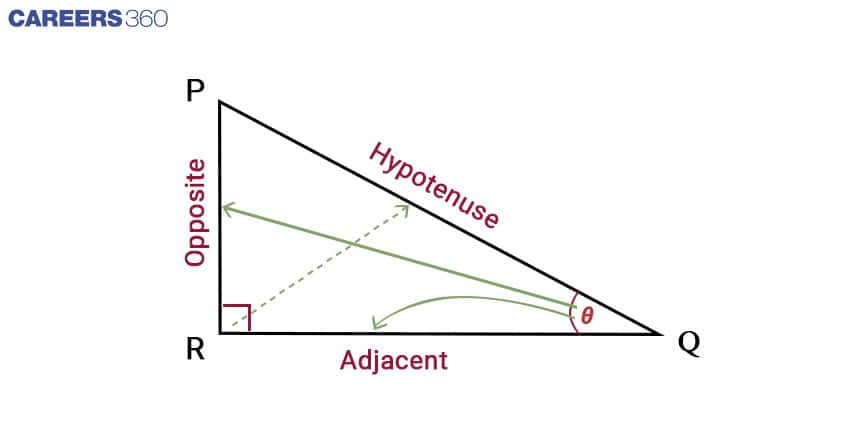With respect to ∠Q, the ratios of trigonometry are given as:

• Sine: It is defined as the ratio of the perpendicular side (side opposite) to that angle to the hypotenuse. This is also commonly called sin.

• Cosine: The ratio of the side adjacent to that angle to the hypotenuse is cosine also called cos.

• Tangent: The ratio of the side opposite to that angle to the side adjacent to that angle is tan or tangent.

• Cosecant: It is a reciprocal of sine. It's also called cosec.

• Secant: It is a reciprocal of cosine.This is commonly known as sec.

• Cotangent: The reciprocal of the tangent is cotangent and is also referred to as cot.

So, for Δ PQR, the ratios are defined as:

• Sin Q = (Opposite side to ∠Q)/(Hypotenuse) = PR/PQ

Trick to remember SOH: S - sin , O - Opposite side , H - Hypotenuse

• Cos Q = (Adjacent side to ∠Q)/(Hypotenuse) = RQ/PQ

Trick to remember CAH: C - cos , A - Adjacent side , H - Hypotenuse

• Tan Q = (Opposite side to ∠Q)/(Adjacent side to ∠Q) = PR/RQ

= sin ∠Q/cos ∠Q

Trick to remember TOA: T- tan , O - Opposite side, A - Adjacent

• cosec Q= 1/sin Q = (Hypotenuse)/ (Side Opposite to ∠Q) = PQ/PR

Trick to remember : Reciprocal of sin

• sec Q = 1/cos Q = (Hypotenuse)/ (Side Opposite to ∠Q) = PQ/RQ

Trick to remember: Reciprocal of cos

• cot Q = 1/tan Q = (Side adjacent to ∠Q)/(Side opposite to ∠Q)= RQ/PR

Trick to remember: Reciprocal of tan

## Easy Tips for Hindi students:

• LAL/KKA, in order to sin, cos, tan

Where,

L - Lambh (Opposite side in Hindi)

K - Karn (Hypotenuse in Hindi)

For above triangle,

Sin Q = L/K = Lambh/ Karn = (Opposite side to ∠Q)/(Hypotenuse) = PR/PQ

Cos Q = A/K= Aadhar/ Karn = (Adjacent side to ∠Q)/(Hypotenuse) = RQ/PQ

Note: We can get the other three ratios by taking the reciprocals of the above three ratios which we have already discussed above.

## Important Fact to know:

In right Δ PQR, if ∠P and ∠Q are assumed as 60° and 30°, then there can be infinite right triangles with those specifications but all the ratios written above for ∠Q in all of those triangles will be same. This means that the ratios are independent of lengths of sides of the triangle.

## Trigonometric Ratios of Some Specific Angles

You are already familiar with the construction of angles of 30°, 45°, 60° and 90° in a triangle. Now, we will learn the values of the trigonometric ratios for these angles 30°, 30°, 60°, 90° and of course, for 0° also.

Values for these triangle is given below in a table:

 ∠θ 0° 30° 45° 60° 90° sin θ 0 1/2 1/√2 √3/2 1 row-1 cos θ 1 √3/2 1/√2 1/2 0 row-2 tan θ 0 1/√3 1 √3 Not defined row-3 cosec θ Not defined 2 √2 2/√3 1 row-4 sec θ 1 2/√3 √2 2 Not defined row-5 cot θ Not defined √3 1 1/√3 0 row-6

## How To Remember The Values Of The Table?

This is a big table and there are so many numbers that's why it is very difficult to memorise all the values for all angles. But we can remember this table in a very simple way by following the instructions given below:

1. To remember all values you just have to remember the order of all angles in the very first column. (sin, cos, tan, cosec, sec, cot) and the values of the first row.

2. To get the second row (all the values for cos), you just have to reverse (from right to left ) the order of the first row.

3. To get the third row (all the values for tan ) just divide the values of the first row by the values of the second row.

4. To get the fourth row just take the reciprocal of the first row.

5. To get the fifth row just take the reciprocal of the second row.

6. To get the sixth row just take the reciprocal of the third row.

## Practice with Previous Years Board Questions

Q-1 Evaluate 2 sec 30° x tan 60° (CBSE 2020)

Solution:

We know that sec 30° = 2/√3 and Tan 60° = √3

Therefore

2Sec 30° x Tan 60° = 2 . 2/√3 . √3 = 4

Hence, the value of 2 sec 30° x tan 60° is equal to 4.

Q-2 If sinA = 1/2 , then value of 2 sinA cosA is ((Rajasthan Board 2022)

Solution

Using the above table we can find that sin 30° = 1/2

Therefore value of A = 30°

Thus 2 sin 30° cos 30°

Again using the table given above we can find that

Sin 30° = 1/2

Cos30° = √3/2

After putting these values in the equation

2 sin 30° cos 30° = 2 (1/2) (√3/2) = √3/2

Hence, value of 2 sinA cosA = √3/2

Also check - What Is The Science Behind Lightning?

## Trigonometric Ratios of Complementary Angles

We know that two angles are known as complementary angles if their sum is equal to 90°. In Δ PQR, right-angled at R. Since P + Q = 90° So, they are complememtry angles.

Now let us write the trigonometric ratios for Q = 90° – P.

sin (90° – Q) = cos Q, cos (90° – Q) = sin Q,

tan (90° – Q) = cot Q, cot (90° – Q) = tan Q,

sec (90° – Q) = cosec Q, cosec (90° – Q) = sec Q,

In general form, for angle θ,

sin (90° – θ) = cos θ, cos (90° – θ) = sin θ,

tan (90° – θ) = cot θ, cot (90° – θ) = tan θ,

sec (90° – θ) = cosec θ, cosec (90° – θ) = sec θ,

For all values of angle θ, between 0° and 90°. Check whether this holds for

θ = 0° or θ = 90°.

We can see tan 0° = 0 = cot 90°, sec 0° = 1 = cosec 90° and sec 90°, cosec 0°. tan 90° and cot 0° are not defined; we can also match this with the table.

## Practice with Previous Years Questions

Q-1 cos80°sin10° + cos 59° cosec 31° = …. (CBSE 2020)

Solution:

We can observe that 80 + 10 = 90 as well as 59 + 31 =90

Therefore we can use concepts of trigonometry angles

sin (90° – θ) = cos θ

sin (90° – 80°) = cos 80°

Sin 10° = cos 80° ………(1)

Also

sec (90° – θ) = cosec θ

sec (90° – 31°) = cosec 31°

sec 59° = cosec 31°

1cos 59° = cosec 31° ……..(2)

Using Equation (1) and (2)

cos 80°sin 10° + cos 59° cosec 31°

= sin 10°sin 10° + cos 59° 1cos 59°

= 1+1 = 2

Hence, cos 80°sin 10° + cos 59° cosec 31° = 2

## Trigonometric Identities

An equation is known as an identity when it is true for all possible values of the variables involved. Similarly, if an equation involving trigonometric ratios is true for all values of the angles involved then it will be known as trigonometric identities. There are three basic trigonometric identities which are given below:

In Δ PQR, right-angled at R.

• cos2 P + sin2P = 1 or cos2 Q + sin2Q = 1

• 1 + tan2 P = sec2 P or 1 + tan2 Q = sec2 Q

• cot2P + 1 = cosec2 P or cot2Q + 1 = cosec2 Q

In general form for angle:

• cos2 θ+ sin2 θ = 1

• 1 + tan2 θ = sec2 θ

• cot2θ + 1 = cosec2 θ , True for all θ such that 0° < θ ≤ 90°.

## Practice with Previous Years Questions

Q-1. If sinθ + cosθ = √3 then prove that tanθ + cot θ = 1(CBSE 2020)

Solution

Given that

sin θ + cos θ = √3

Square the LHS and RHS

cos2 θ+ sin2 θ +2 sinθ cosθ = 3

We know that cos2 θ+ sin2 θ = 1

Using the above equation we can say that sinθ cosθ = 1

We want to prove tanθ + cot θ = 1

We know that tanθ = sinθcosθ and cotθ = cosθsinθ

LHS = tanθ + cot θ = sinθ/cosθ + cosθ/sinθ = (sin2θ + cos2θ)/(sinθ cosθ) = 1/1 = 1 =RHS

Hence proved

## Some Applications of Trigonometry

Trigonometry was discovered because of its need in astronomy. Astronomers have used it to calculate distances from the earth to the stars and other planets. It is also used in navigation and geography.

Trigonometry is also used to construct maps, determine the position of an island, to calculate the longitudes and latitudes for an object, to calculate height and distance of an object, to calculate the distance between two objects, etc.

• Mathematics
• Mathematical objects
• Geometry
• Mathematical analysis
• Elementary mathematics
• Complex analysis
• Elementary geometry
• Geometric measurement
• Trigonometry
• Trigonometric Functions

## Careers360 helping shape your Career for a better tomorrow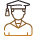#### 250M+

Students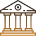#### 30,000+

Colleges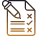#### 500+

Exams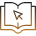E-Books